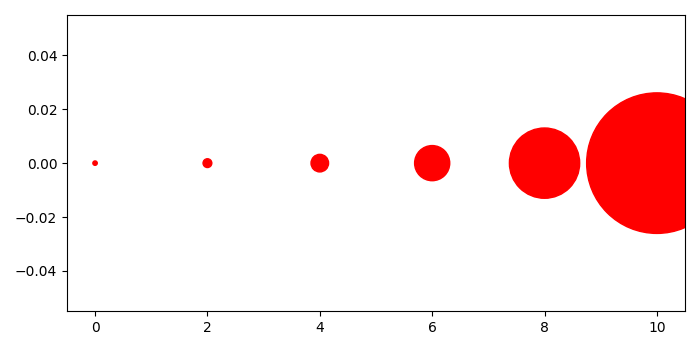# How to plot scatter points with increasing size of marker in Matplotlib?

To plot scatter points with increasing size of marker, we can take the following steps−

## Steps

• Create x and y data points
• To get increasing size of marker, make a list of numbers.
• Use scatter method to plot scatter points.
• To display the figure, use show() method.

## Example

from matplotlib import pyplot as plt
plt.rcParams["figure.figsize"] = [7.00, 3.50]
plt.rcParams["figure.autolayout"] = True
x = [0, 2, 4, 6, 8, 10]
y =  * len(x)
s = [10 * 4 ** n for n in range(len(x))]
plt.scatter(x, y, s=s, c='red')
plt.show()

## Output